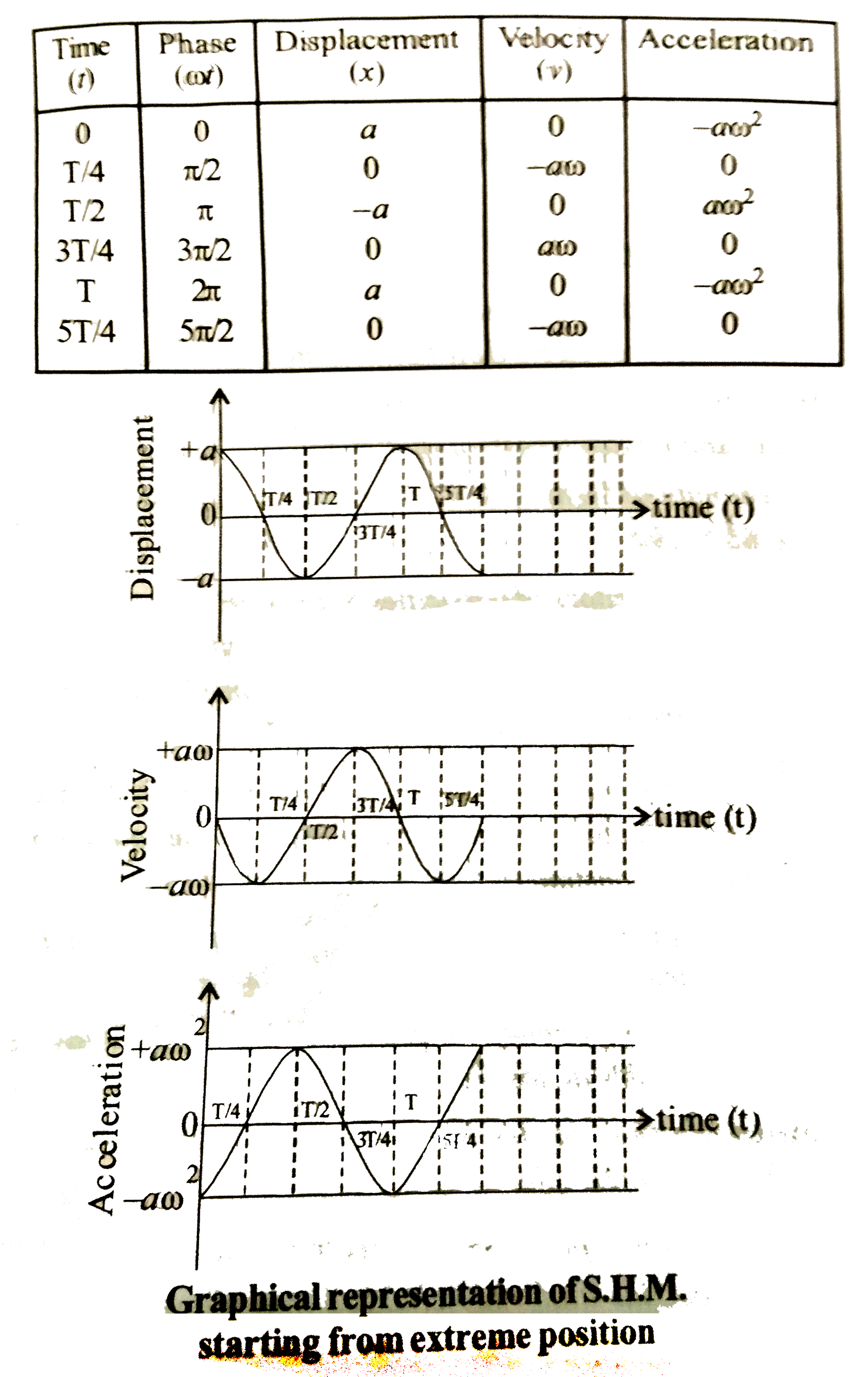# Define linear simple harmonic motion. Assuming the expression for displacement of a particle starting from extreme position, explain graphically the v

476 views
in Physics
closed
Define linear simple harmonic motion. Assuming the expression for displacement of a particle starting from extreme position, explain graphically the varition of velocity and acceleration w.r.t. time.
A clock reagulated by seconds pendulum, keeps correct time. During summer, length of pendulum increases to 1.005 m. How much will the clock gain or loose in one day (g=9.8m//s^(2)andpi=3.142).

by (91.7k points)
selected

Linear Simple Harmonic Motion : It is defined as the linear periodic motion of a body, in which the restoring force (or acceleration) is always directed towards the mean position and its magnitude is directly proportional to the displacement from the mean position.
Particle performing S.H.M. starting fromm extreme position :
Equation of displacement is x=acosomegat
At extreme position, alpha=pi//2.
:. Velocity of particle is v=(dx)/(dt)=(d)/(dt)(acosomegat)
And v=-aomegasinomegat
acceleration of a particle =(dv)/(dt)
=(d)/(dt)=(-aomegasinomegat)
=-aomega^(2)cosomegat.
Substiuting omega=2pi//T in above equaitons(i) Displancement, veloctiy and acceleration of S.H.M. are periodic functions of time.
(ii) Displancement-time curve and acceleration-time curve are cosine curve and velocity-time curve is a since curve.
(iii) Phase difference between displacement and velocity is
(pi)/(2) radian.
(iv) Phase difference between velocity and acceleration is (pi)/(2) radian.
(v) Phase difference between displacement and acceleration is pi radian.
(vi) At extreme position, displancement and acceleration is maximum while velocity is minimum.
(vii) After the phase of 2pi radian, all curves repeat the same path.
Given L=1.005m and g =9.8m//s^(2)
Period of simple pendulum (T)
=2pisqrt((L)/(g))
T=2xx3.142xxsqrt((1.005)/(9.8))
=2.012sec.
Now, seconds pendulum has a period of 2 sec.
Therefore, the given clock will loose (2.012-2)sec=0.012 sec during summer.
:. Loss in period per day (24xx3600xx0.012)/(2.012)
=515.3` sec.

+1 vote